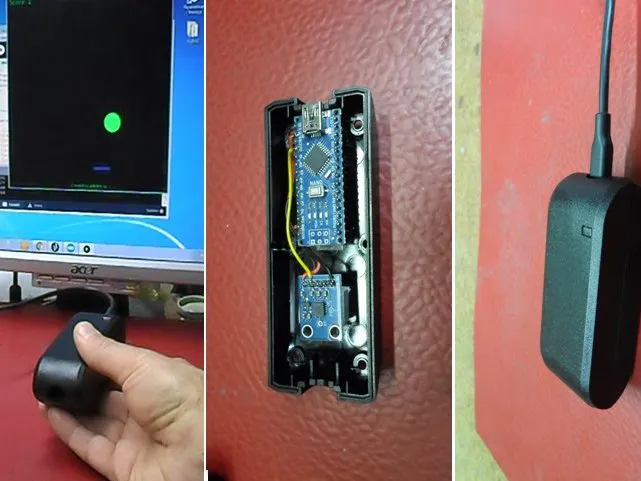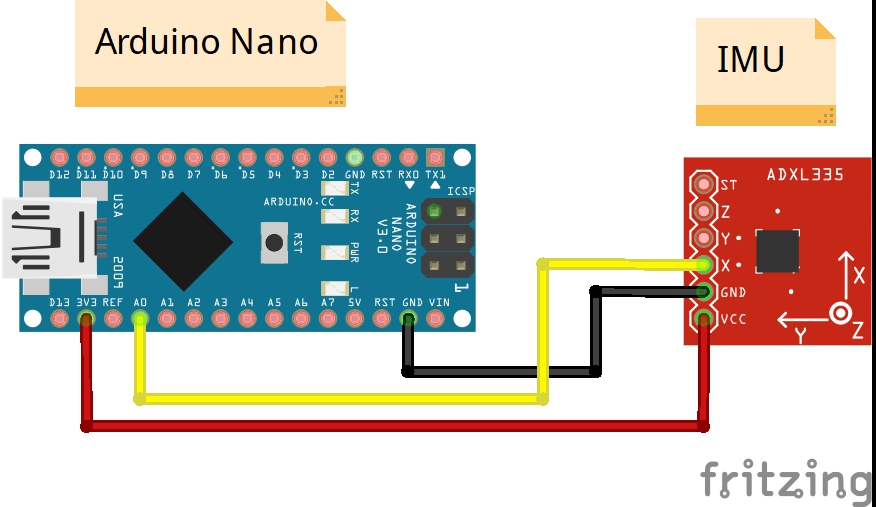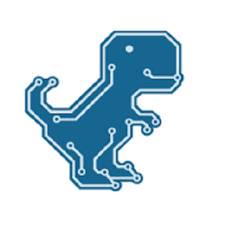Project tutorial# Interactive Pong PC Game with ADXL335 Accelerometer © GPL3+

The classic PC game Pong using an Arduino and an accelerometer.

• 1,495 views
• 0 comments
• 4 respects

## Necessary tools and machinesSoldering iron (generic)

## About this project

Augmented reality and virtual gaming has become a recent trend in the gaming industry. The times of using a keyboard/Joystick and a mouse to play a computer game has gone behind. Now every gaming console comes with a virtual controller that helps us to play the game using our body movements and gestures, this way the gaming experience has increased a lot and user feels more involved into the game. On "circuitdigest" page is presented an example that use Arduino Nano and ADXL335 sensor board.

The ADXL335 is a small, thin, low power, complete 3-axis accelerometer with signal conditioned voltage outputs. In this case we will only use one axle (x) which we need to move the bumper left and right. The accelerometer is connected to the Arduino using only 3 wires as shown in the scheme below. It is powered directly from the Arduino USB port.

The software part consists of Arduino and Processing code.The Arduino program is given below in the Code section. There is no critical data that has to be modified. But you might want to consider the following:

Increase the sample size if your Accel still shows random values:

#define Samplesize 13 // filterSample number

In the void setup() function of Processing code is important, as it decides from which port to data from.

port = new Serial(this, Serial.list(), 9600); //Reads the 4th PORT at 9600 baudrate

Now since our Processing and Arduino sketch is ready, just upload the below given program to Arduino and connect your Arduino to user PC thorough programming cable and launch the game by running the Processing code file (.pde). That's it! Move your accelerometer and play your Pong game.

## Code

##### Arduino codeC/C++
```/*
* Program for filtering the X-axis values of accel and transmitting it serially
* Programmed by B.Aswinth Raj
* Dated: 21-08-206
*/

#define AccelPin      A0         // A0 is connected to X-axis of Accel
#define Samplesize   13         // filterSample number
int Array1 [Samplesize];           // array for holding raw sensor values for sensor

int rawData1, smoothData1;      // variables for sensor data

int toSend;

void setup(){
Serial.begin(9600);
}

void loop()
{
rawData1 = analogRead(AccelPin);                              // read X-axis of accelerometer
smoothData1 = digitalSmooth(rawData1, Array1);

toSend = map (smoothData1, 193, 280, 0, 255);             // the data from accelerometer mapped to form a byte
Serial.write (toSend);
delay(100);
}

int digitalSmooth(int rawIn, int *sensSmoothArray){         // "int *sensSmoothArray" passes an array to the function - the asterisk indicates the array name is a pointer
int j, k, temp, top, bottom;
long total;
static int i;
static int sorted[Samplesize];
boolean done;

i = (i + 1) % Samplesize;                  // increment counter and roll over if necc. -  % (modulo operator) rolls over variable
sensSmoothArray[i] = rawIn;           // input new data into the oldest slot

for (j=0; j<Samplesize; j++){           // transfer data array into anther array for sorting and averaging
sorted[j] = sensSmoothArray[j];
}

done = 0;                    // flag to know when we're done sorting
while(done != 1){        // simple swap sort, sorts numbers from lowest to highest
done = 1;
for (j = 0; j < (Samplesize - 1); j++){
if (sorted[j] > sorted[j + 1]){        // numbers are out of order - swap
temp = sorted[j + 1];
sorted [j+1] =  sorted[j] ;
sorted [j] = temp;
done = 0;
}
}
}

bottom = max(((Samplesize * 15)  / 100), 1);
top = min((((Samplesize * 85) / 100) + 1  ), (Samplesize - 1));   // the + 1 is to make up for asymmetry caused by integer rounding
k = 0;
total = 0;
for ( j = bottom; j< top; j++){
total += sorted[j];         // total remaining indices
k++;

}

return total / k;            // divide by number of samples
}
```
##### Processing codeC/C++
```/*
Program to create a game and gets its motion values serially from 3rd PORT
Programed by B.Aswinth Raj
Dated on : 21-08-2016
*/

import processing.serial.*;

Serial port;

int data;
int movby;
float x=300;
float y=00;
float spdy=6;
float spdx=0;
float posxr,posxl,posyr,posyl,ballpos;
int score = 0;
float speed=0;
float flag=0;
float ballsize=20;
float temp;

void setup()
{
size(600,600,P2D);
smooth();
port = new Serial(this,Serial.list(),9600);
println(Serial.list());
}

void draw()
{
if (port.available()>0 && port.available()<30)
{
data=port.read();
movby = int(map(float(data), 255,0,30,570));
println(movby);
}

if (flag==0)
strtscrn();
if (flag==1)
game();
if (flag==3)
lastscrn();

fill(18,250,8);
textSize(12);
text("Created by asWINth raj", 245, 590);

textSize(22);
text("Score:",10,20);
text(score, 80, 20);
fill(0, 102, 153);
}

void strtscrn()
{score=0;
cursor();
background(255); //Color of the backgroud
fill(18,250,8);
textSize(52);
text("WELCOME", 200,300);
fill(18,250,80);
textSize(32);
text("Select your Level", 200,330);
fill(180,250,8);
textSize(22);
text("1   2    3   4", 250,370);
//println(mouseX, mouseY);
if (mouseY > 340 && mouseY < 380)
{
cursor(HAND);
if(mousePressed==true && flag==0)
{

if (mouseX >240 && mouseX <270)
{
speed=1;
}
if (mouseX >280 && mouseX <300)
{
speed=2;
}
if (mouseX >320 && mouseX <350)
{
speed=3;
}
if (mouseX >350 && mouseX <380)
{
speed=4;
}
flag=1;
}

}

}

void game()
{
noCursor();
background(0); //Color of the backgroud
y=y+spdy; //speed and positon of ball in Y axis
x=x+spdx; //speed and positon of ball in X axis

rectMode(CENTER);
fill(16,22,162);
rect(movby,530,60,10); //The plate

posxr=movby+40;
posxl=movby-40;
posyr=530+15;
posyl=530-10;

if (( (posyl < y) && (y < posyr) ) && ( (posxl < x) && (x < posxr) ))  //Plate and ball meeting
{
spdy=-(speed*3);

if (x<movby) //Pad left deflection
{
ballpos=movby-x;
spdx=-(ballpos/5);
// println(ballpos);
}

if (x>movby)  //Pad right deflection
{
ballpos=x-movby;
spdx=+(ballpos/5);
//println(ballpos);
}

}

if (x<=0 ) // Left margin deflection
spdx=(speed*3);

if (y<=0 ) // Top margin deflection
{
spdy=(speed*3);
score=score+1;
}

if (x>=600) // Right margin deflection
spdx=-(speed*3);

if (y>=600)
{
background(250,0,0);
x=300;
y=0;
flag=3;
speed=1;
}

fill(88,250,68);
ellipse(x,y,ballsize,20); //The ball

if(score>=15 && score<=20)
{
ellipse(random(600),random(600),20,20);
ellipse(random(600),random(600),20,20);
ellipse(random(600),y,20,20);
ellipse(x,random(600),20,20);///The ball
}

temp=x;

}

void lastscrn()
{
background(250,0,0);
textSize(32);
text("GAME OVER", 200,300);
fill(18,250,80);
textSize(22);
text("Your Score:", 200,330);
text(score, 320,330);
fill(180,250,8);

text("Click to try again..", 200,370);
if(mousePressed == true)
flag =0;
}
```

## Schematics## Comments

#### Author##### Mirko Pavleski
• 47 projects
• 164 followers

#### Published on

November 23, 2019

#### Members who respect this project

See similar projects
you might like

#### DIY Sensitive ADXL335 Earthquake Detector

Project tutorial by Mirko Pavleski

• 3,123 views
• 3 comments
• 9 respects

#### Arduino Pong Game on 24x16 Matrix with MAX7219

Project tutorial by Mirko Pavleski

• 3,363 views
• 3 comments
• 19 respects

#### Arduino Gyroscope Game with MPU-6050

Project tutorial by Mirko Pavleski

• 2,958 views
• 2 comments
• 12 respects

#### 8×8 LED and Accelerometer

Project tutorial by Team Brink.IO

• 2,073 views
• 0 comments
• 4 respects

#### Level Platform Using Accelerometer

Project tutorial by Mark Tashiro

• 25,101 views
• 19 comments
• 38 respects

#### Gesture Control Robot

Project tutorial by Raunak Oberai

• 15,381 views
• 18 comments
• 37 respects